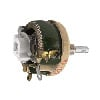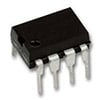# Variable Resistor

## What is a Variable Resistor?

A variable resistor is a resistor of which the electric resistance value can be adjusted. A variable resistor is in essence an electro-mechanical transducer and normally works by sliding a contact (wiper) over a resistive element. When a variable resistor is used as a potential divider by using 3 terminals it is called a potentiometer. When only two terminals are used, it functions as a variable resistance and is called a rheostat. Electronically controlled variable resistors exist, which can be controlled electronically instead of by mechanical action. These resistors are called digital potentiometers.

## Variable Resistor Definition

A resistor of which the ohmic resistance value can be adjusted. Either mechanically (potentiometer, rheostat) or electronically (digital potentiometer).

## Types of Variable Resistors

### PotentiometerThe potentiometer is the most common variable resistor. It functions as a resistive divider and is typically used to generate a voltage signal depending on the position of the potentiometer. This signal can be used for a very wide variety of applications including: amplifier gain control (audio volume), measurement of distance or angles, tuning of circuits, and much more. When variable resistors are used to tune or calibrate a circuit or application, trimmer potentiometers or trimpots are used. These are most often small potentiometers mounted on the circuit board and can be adjusted using a screwdriver.

### RheostatRheostats are very similar in construction to potentiometers, but are not used as a potential divider, but as a variable resistance. They use only 2 terminals instead of the 3 terminals that potentiometers use. One connection is made at one end of the resistive element, the other at the wiper of the variable resistor. In the past, rheostats were used as power control devices in series with the load, such as a light bulb. Today, rheostats are not often used for power control because this is an inefficient method. For power control, rheostats have been replaced by more efficient switching electronics. Preset variable resistors, wired as rheostats, are used in circuits to perform tuning or calibration.

### Digital resistorA digital variable resistor is a type of variable resistor where the change of resistance is not performed by mechanical movement but by electronic signals. They can change resistance in discrete steps and are often controlled by digital protocols such as I2C or by simple up/down signals.

## Symbols for Variable Resistors

The following figure provides the IEC standard symbols for three types of variable resistors.Potentiometer symbolRheostat symbolPreset resistor symbol